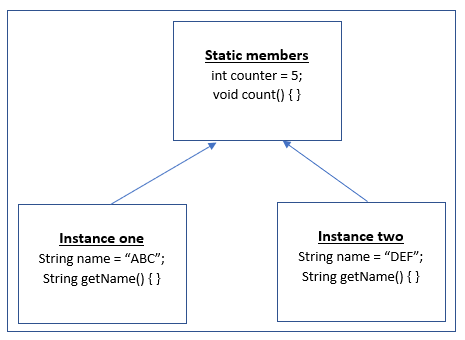## static keyword in java

static keyword
`static` is a reserved word(or keyword) in java. It can be applied before a

• field(or instance member)
• method
• class
• unnamed block

Writing `static` keyword before any of the above makes it static.

static meaning in java
`static` means belonging to the class and not to the instance. If a field, method or a block is made `static`, then it is shared by all the instances(or objects) of the class. This also means that only one copy of the `static` member exists for all instances.
Remember that `static` members of a class exist independently before even any instance of the class is created.
Suppose there is a class which contains both `static` and non-static fields and methods.

```public class StaticExample {   // non-static field private String name;   // non-static field private int age;   // static field public static int counter;   // instance method public String getName() { return name; }   // instance method public void setName(String name) { this.name = name; }   // static method public static void count() { counter++; }   // Overridden toString method public String toString() { return "[Name= "+name+", age= "+age+"]"; } }```

If a couple of instances of the above class are created, then they will be represented in memory asNote that all the instances share the `static` members and if one instance of a class modifies the value of a `static` variable, then it is visible across all the instances of that class.

As stated earlier, `static` members(variables and methods) are directly linked to the class and not to any instance, thus they can be accessed directly using the class name.
In other words, no instance of a class is required to access its `static` members. Thus, in above example, `static` members can be accessed as

StaticExample.counter;
StaticExample.count();

where `StaticExample` is the name of class.
Use of static keyword in java
`static` variables and methods are most commonly used when they should not have any relation to an instance of a class.
Let’s say a variable should hold some information that is common for all objects or a method that performs its task with that task having no association with the class objects, then it is better to make them `static`.
Below scenarios will be ideal examples for the use of `static` variable and method in java.

1. Suppose a method should return the current system time or system IP address, it does not make any sense to bind it to an instance since these attributes will be common for all objects.
2. A variable that holds the number of objects of the class being created. In this case, you need a common variable that should be incremented when a new object is created.
It can not be linked with any particular instance.
3. A method that simply prints a message to the console. It would be nice to make it common for all objects since its only task is to output data without any relation to any object.

Where as, suppose a variable should hold the name of a person. Now each person is represented by a separate instance(or object) and hence would have a different name.
Thus, it should not be made a `static` field but a non-static or instance variable.
static example in java

Below is an example of using `static` and non-static variables and methods in java. It will be using the `StaticExample` class defined above.

```public class StaticDemo { public static void main(String[] args) { // create object of class StaticExample objectOne = new StaticExample(); objectOne.name = "ABC"; objectOne.age = 23; // create second object of class StaticExample objectTwo = new StaticExample(); objectTwo.name = "DEF"; objectTwo.age = 45; // access static method StaticExample.count(); // access and print static variable System.out.println(StaticExample.counter); // print objects System.out.println(objectOne); System.out.println(objectTwo); } }```

Above program creates two objects and initializes their name and age fields. It also calls its `static` method which increments the value of `static` variable.
Finally, it prints the value of `static` variable and prints the objects created. Notice the difference between the way `static` and non-static fields are accessed.
Output
Above program will produce below output.

1
[Name= ABC, age= 23]
[Name= DEF, age= 45]

Also, static fields should be accessed using class name only and not using objects.

Though you can access it using instance variable also but it is not recommended and the compiler will flag a warning such as

The static field StaticExample.counter should be accessed in a static way

This is because `static` members do not belong to any particular object or instance.
Non permissible static usage
Following can not be marked `static` in java

• Class(except nested class),
• Interface,
• Local variables,
• Constructors,
• Method local inner class.

Using `static` before the above will be a compiler error.

Click the clap if the article was helpful for you.

0